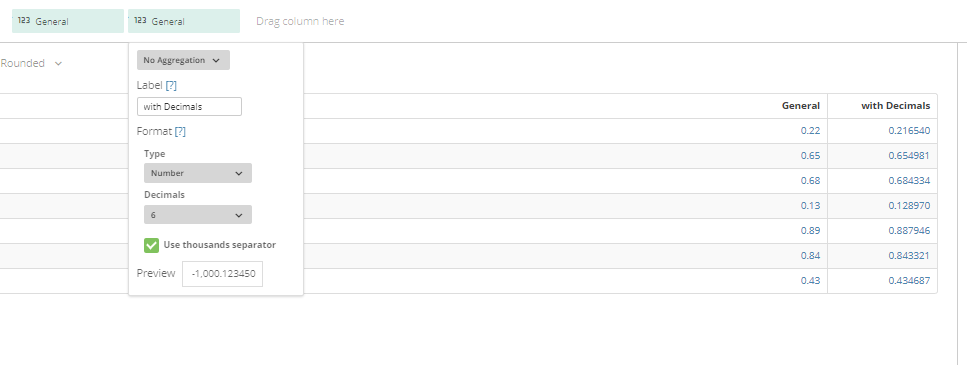# How do I avoid decimals from being truncated while bringing data from Excel?

I have an Excel that has several measures that are percentages.  The Excel is in a Box folder, so I am using the Box connector to bring the data to a Domo dataset.  Everything works fine, except that the percentages get converted to decimal numbers (so, all are <1) and truncated to 2 decimals... so that is causing me issues when I use the SUM option for those percentages in my visualizations.

How do I fix it?  Thanks!

• The values are still there.  They have just been imported as type DOUBLE.  Depending on what you are wanting to do with the data, you can either convert it in MySQL using something like this:

SELECT convert (`General`, decimal(9,7)) as `General` from dojo_help_2

You can use an ETL by usint the "Set Column Type" action and changing the data type to Decimal(Fixed)

You can just change the chart options to display it as a number with x decimals: